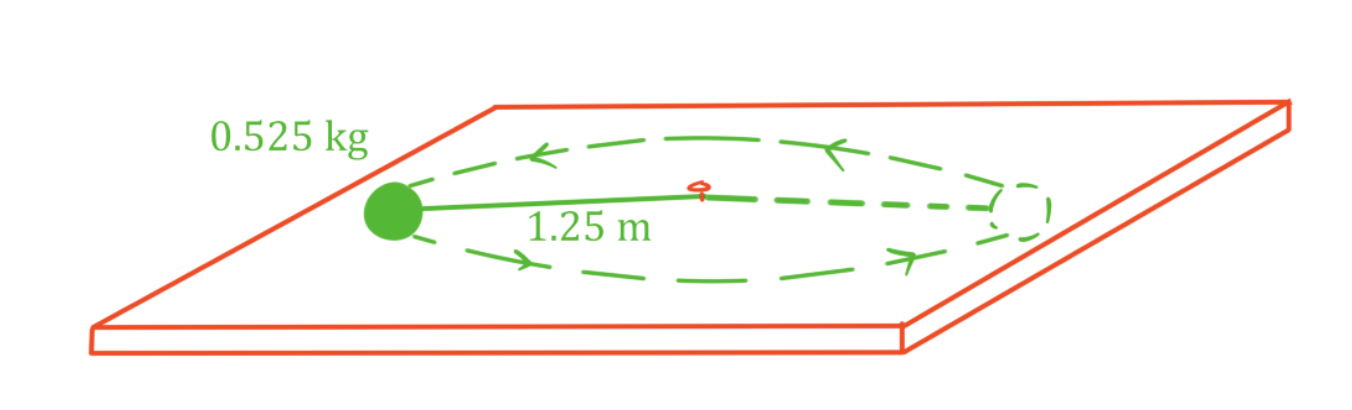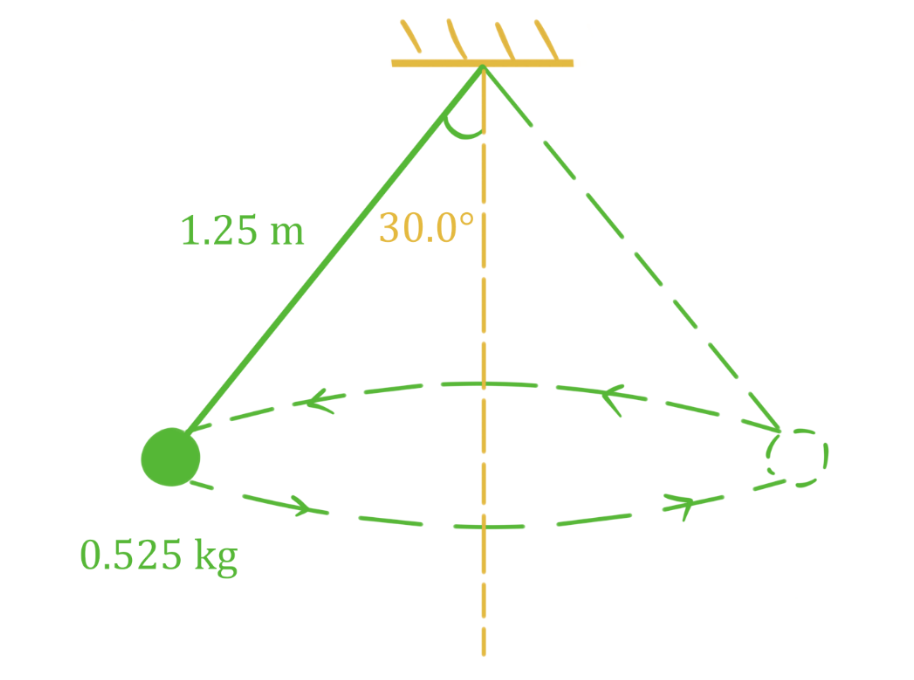# Horizontal circular motion#### Everything You Need in One Place

Homework problems? Exam preparation? Trying to grasp a concept or just brushing up the basics? Our extensive help & practice library have got you covered.#### Learn and Practice With Ease

Our proven video lessons ease you through problems quickly, and you get tonnes of friendly practice on questions that trip students up on tests and finals.#### Instant and Unlimited Help

Our personalized learning platform enables you to instantly find the exact walkthrough to your specific type of question. Activate unlimited help now!##### Examples
###### Lessons
1. Ball on horizontal string moving in circular motion

A 0.525 kg ball is attached to a 1.25 m string and swings in a circular path, making 2.00 revolutions per second. The angle of the string is nearly horizontal. Find the tension in the string.1. Ball on angled string in horizontal circular motion

A 0.525 kg ball is attached to a 1.25 m string and swings in a circular path. The angle of the string away from vertical is 30.0°. Find the centripetal force acting on the ball and the speed of the ball.###### Topic Notes
In this lesson, we will learn:
• Meaning of uniform circular motion
• Solving problems involving horizontal circular motion

Notes:

• An object moving in a circular path is in circular motion. If the speed of the object is constant, it is uniform circular motion.
• An object in uniform circular motion does experience acceleration, even though its speed is constant. Remember, acceleration is change in velocity, and a velocity is made up of speed and direction. For the object to move in a circle, the direction of its velocity must change constantly. This change in direction is the acceleration, called centripetal acceleration ("centripetal" means "towards the center"). For an object moving in a circular path, the centripetal acceleration vector is always pointed towards the center of the circle.
• Like any other type of acceleration, centripetal acceleration is caused by a force (called centripetal force). The centripetal force vector also always points towards the center of the circle.
• In order for an object to be moving in a circular path, the net force acting on the object must be a centripetal force (a force that always is pointed towards the center). When multiple forces act on an object in circular motion, those forces must add up to a centripetal force. It is important to understand that centripetal force is not a separate force that acts on an object. It is a net force which follows a specific rule: it always points towards the center of the circular path.
• In a horizontal circular motion problem, any forces acting on the object in the vertical direction must balance so that $\Sigma F_{vertical} = 0N$ (otherwise the object would accelerate vertically). Only horizontal forces will contribute to the net force causing circular motion.
Period and Frequency

$T = \frac{total time}{\# of revolutions}$

$f = \frac{\# of revolutions}{total time}$

$T = \frac{1}{f}$

$T:$ period, in seconds (s)

$f:$ frequency, in hertz (Hz)

Centripetal Acceleration

$a_{c} = \frac{v^{2}}{r} = \frac{4\pi^{2}r}{T^{2}}$

$a_{c}:$ centripetal acceleration, in meters per second squared $(m/s^{2})$

$v:$ velocity, in meters per second (m/s)

$r:$ radius, in meters (m)

$T:$ period, in seconds (s)